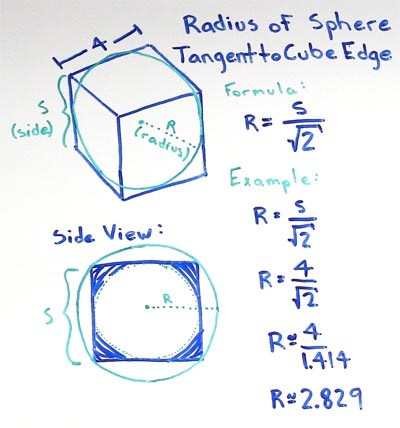# Volume of Cube

## - Sphere Radius Tangent to Edge of Cube

A cube with a side length (S) of 5 has a tangent sphere with a radius (R) of 3.5355.

for cubes with a side length S find the radius R of the sphere tangent to the cube edges

• R = S/√2

substitute the side length S with the measured value, in this example lets use a side length of 5

• R = 5/√2

divide the side length by the square root of 2 to find the radius of a sphere tangent to the edges of the cube

• R = 3.5355Given a cube with a side length S the radius (R) of a sphere tangent to the cube edges can be found by dividing the cube side length by the square root of two (2).

### Formulas

What is the formula for the volume of a Cube?

V = S3

What is the formula for the volume of a Rectangular Prism?

V = L x W x H

### Other Formulae

for cubes with side length S

Cube Surface Area

6S2
Cube Face Diagonal
(√2)S
Cube Space Diagonal
(√3)S
S/2
All formulae...